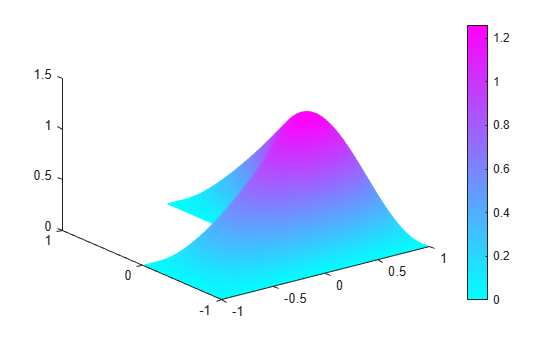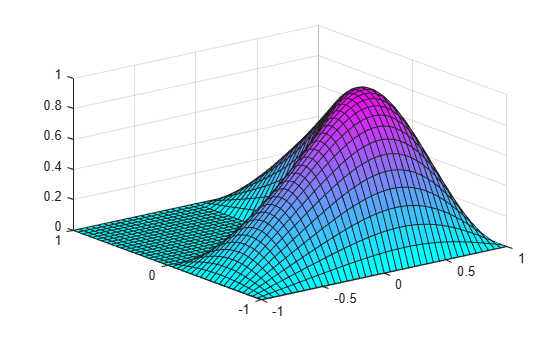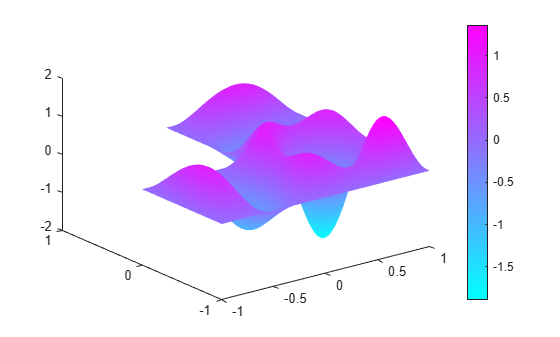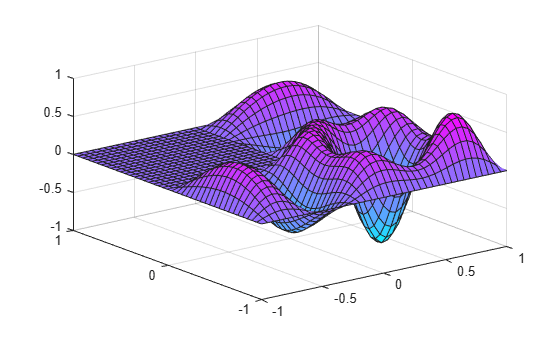# Eigenvalues and Eigenmodes of L-Shaped Membrane

This example shows how to calculate eigenvalues and eigenvectors. The eigenvalue problem is $-\Delta u=\lambda u$. This example computes all eigenmodes with eigenvalues smaller than 100.

Create a model and include this geometry. The geometry of the L-shaped membrane is described in the file `lshapeg`.

```model = createpde(); geometryFromEdges(model,@lshapeg);```

Set zero Dirichlet boundary conditions on all edges.

```applyBoundaryCondition(model,"dirichlet", ... "Edge",1:model.Geometry.NumEdges, ... "u",0);```

Specify the coefficients for the problem: `d` = 1 and `c` = 1. All other coefficients are equal to zero.

`specifyCoefficients(model,"m",0,"d",1,"c",1,"a",0,"f",0);`

Set the interval `[0 100]` as the region for the eigenvalues in the solution.

`r = [0 100];`

Create a mesh and solve the problem.

```generateMesh(model,"Hmax",0.05); results = solvepdeeig(model,r);```

There are 19 eigenvalues smaller than 100.

`length(results.Eigenvalues)`
```ans = 19 ```

Plot the first eigenmode and compare it to the MATLAB's `membrane` function.

```u = results.Eigenvectors; pdeplot(model,"XYData",u(:,1),"ZData",u(:,1));``````figure membrane(1,20,9,9)```Eigenvectors can be multiplied by any scalar and remain eigenvectors. This explains the difference in scale that you see.

`membrane` can produce the first 12 eigenfunctions for the L-shaped membrane. Compare the 12th eigenmodes.

```figure pdeplot(model,"XYData",u(:,12),"ZData",u(:,12));``````figure membrane(12,20,9,9)```